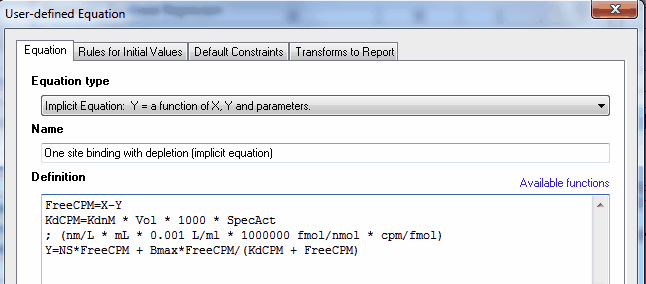## Please enable JavaScript to view this site.

 Entering an implicit equation

An implicit equation is one where Y appears on both sides of the equals sign. Prism will (try to) fit models defined by implicit equations to your data.

## Example of an implicit equation

The model for total binding at equilibrium to a binding site that follows the law of mass action is

Y = NS*X + Bmax*X/(Kd + X)

That form of the model assumes that X is the free concentration of ligand. Since X is actually the concentration you entered, this model assumes that a tiny fraction of the ligand binds so even though X is concentration you applied it also is very close to the free concentration.

What if a substantial fraction of the ligand binds? A much more complicated model is built into Prism to handle this situation. But in other similar situations, the model may not have been derived and it may be very difficult or even impossible to derive one. Implicit equations to the rescue.

In the situation above, it is easy to write an implicit equation. There are three places in the model where X appears. The X values you enter are the total concentration of ligand. The value that enters into the model must be the free concentration, which is the total concentration minus the concentration that bound. Assuming X and Y are both in the same units, we simply replace X with X-Y in all three places:

Y = NS*(X-Y) + Bmax*(X-Y)/(Kd + (X-Y))

When you actually go to fit the data, you'll probably want to change the equation a bit (as shown below) to handle unit conversions so the Kd is reported in nM rather than cpm.

## How to fit implicit equations with Prism

Choose the implicit equation type at the top of the Equation dialog, and define the equation with Y on both sides of the equals sign.That's it. You'll need to define constraints and initial values as with any user-defined equation.

## Notes

In this example you don't actually see Y on both sides of the equals sign in the same equation line. But the first line puts Y on the right side of the equations sign, and the fourth line puts it on the right side. That makes the equation implicit.

If you subtract Y from X, as in the example here, then X and Y must both be entered in the same units. Here both are entered as radioactivity counts per minute (cpm). Of course, it would make no sense to subtract Y from X if Y were in cpm and X were in nM.

Prism finds it "harder" to fit implicit equations than ordinary ones. You may have to fuss with initial values and constraints to get it to work. The calculations take much longer, although this may not be noticeable with small data sets and fast computers.

While Y appears on both sides of the equals sign in an explicit equation, X must appear only on the right side of the equals sign.

In this particular example, the explicit equation has been derived and is even built into Prism. The file you can download (link below) fits the data both ways (explicit equation and implicit equation) and the results are identical. In other cases, it may be difficult or impossible to derive an explicit equation.

Example Prism file.